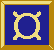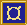# ¤ hSplitF', vSplitF', et al

Containers

## Types

```hSplitF' :: Double -> F a1 a2 -> F c b -> F (a1 ⊕ c) (a2 ⊕ b)
vSplitF' :: Double -> F a1 a2 -> F c b -> F (a1 ⊕ c) (a2 ⊕ b)
splitF' :: LayoutDir -> Double -> F a1 a2 -> F c b -> F (a1 ⊕ c) (a2 ⊕ b)```

## Synopsis

`hSplitF' alignment fud1 fud2`

## Description

These combinator creates horizontal/vertical compositions of fudgets where the amount of space assigned to the parts is controlled by the user.

### Input

Propagated to fud1 or fud2 as in a tagged parallel composition

### Output

the tagged output from fud1 and fud2 as in tagged parallel composition

## Arguments

`alignment :: Double`
determines where the extra space goes when the window is enlarged. aLeft/aTop means that the split position follows the left/top edge of the window. aRight/aBottom analogously means that the split point follows the right/bottom edge of the window. aCenter means that the extra space is divided equally between the two parts.

`fud1 :: F a1 a2`
The first fudget
`fud2 :: F c b`
The second fudget

## Equalities

```  hSplitF' = splitF' Horizontal
vSplitF' = splitF' Vertical
```